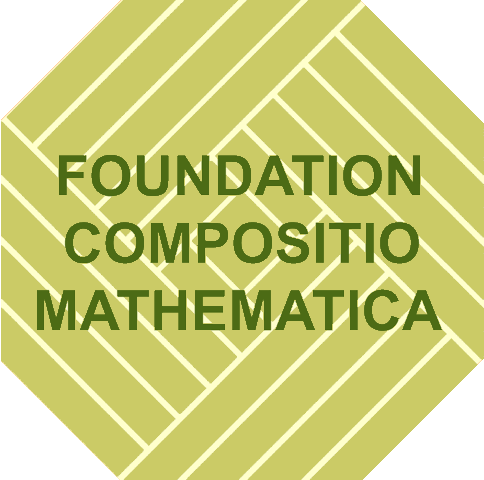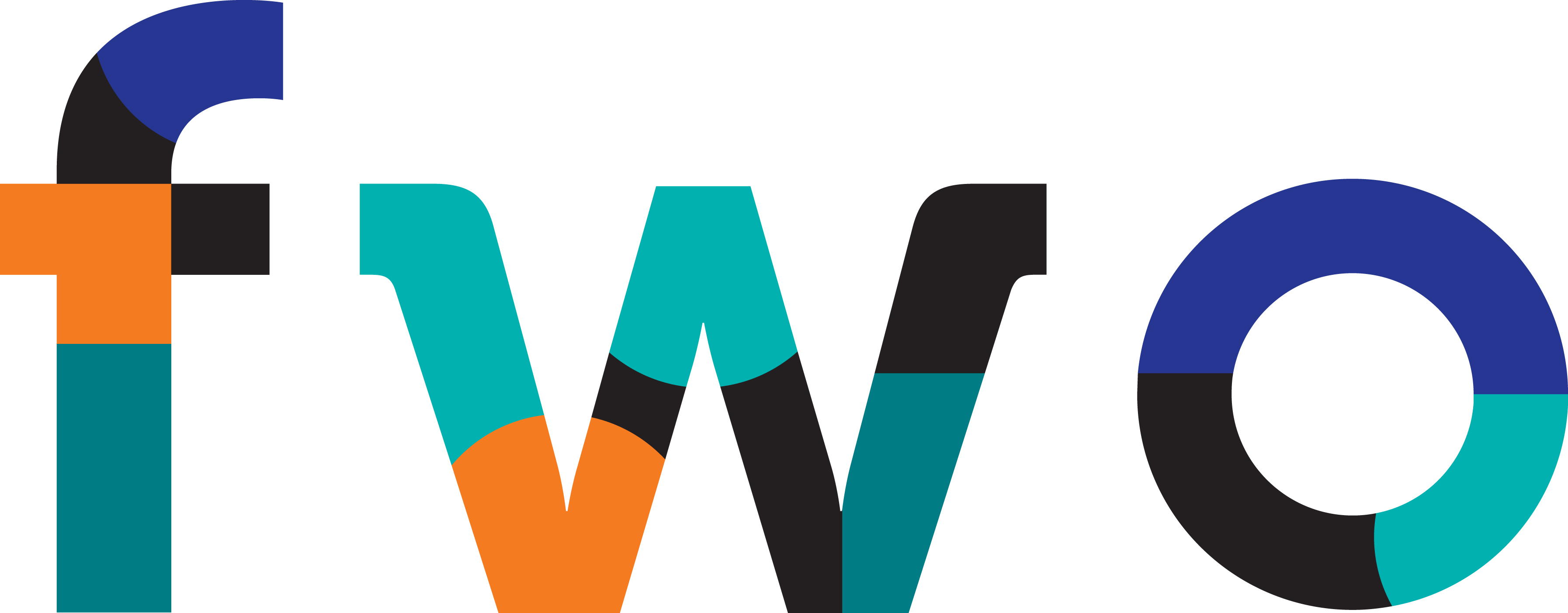### Workshop Goals

This workshop is a forum of mathematicians, computer scientists, engineers and physicists performing research on finite field arithmetic, interested in communicating the advances in the theory, applications, and implementations of finite fields. The workshop will help to bridge the gap between the mathematical theory of finite fields and their hardware/software implementations and technical applications.

### WAIFI 2016 took place from July 13--15, 2016, at Ghent University.

The local host was the Department of Mathematics of Ghent University

The topics of WAIFI 2016 include but are not limited to:

• Theory of finite field arithmetic:

• Bases (canonical, normal, dual, weakly dual, triangular ...)
• Polynomial factorization, irreducible polynomials
• Primitive elements
• Prime fields, binary fields, extension fields, composite fields, tower fields ...
• Elliptic and Hyperelliptic curves

• Hardware/Software implementation of finite field arithmetic:

• Optimal arithmetic modules
• Design and implementation of finite field arithmetic processors
• Design and implementation of arithmetic algorithms
• Pseudorandom number generators
• Hardware/Software Co-design
• IP (Intellectual Property) components
• Field programmable and reconfigurable systems

• Applications:

• Cryptography
• Communication systems
• Error correcting codes
• Finite geometry
• Quantum computing

### Talks

Almost all slides have been collected and are available online at the Program section, by clicking on the title.